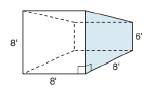Chapter 9.1, Problem 30EElementary Geometry For College St...

7th Edition
Alexander + 2 others
ISBN: 9781337614085

Solutions

Chapter
SectionElementary Geometry For College St...

7th Edition
Alexander + 2 others
ISBN: 9781337614085
Textbook Problem

A storage shed is in the shape of a trapezoidal prism. Each trapezoid represents one of its bases. With dimensions as shown, what is the storage capacity (volume) of its interior?To determine

To find:

The storage capacity (volume) of the interior of the storage shed in the shape of a trapezoidal prism.

Explanation

The volume of any solid is the region of space occupied by it and in general the volume of a solid is given by the product of its base area and altitude.

For a regular solid prism with base area B and altitude h, the volume is given by the formula

V=Bh.

Calculation:

Given,

The volume of the a prism with base area ‘B’ and height ‘h’ is given by the formula.

V=Bh

First let us calculate the base area of the prism.

The base of the prism is trapezoidal and its area is given by the formula.

B=12h(a+b)

Here, ‘a’ and ‘b’ are the length of the parallel sides of the trapezium and ‘h’ is the altitude of the trapezium.

From the given figure we have,

a=8 in

Still sussing out bartleby?

Check out a sample textbook solution.

See a sample solution

The Solution to Your Study Problems

Bartleby provides explanations to thousands of textbook problems written by our experts, many with advanced degrees!

Get Started

Convert the expressions in Exercises 6584 to power form. 18xx23x35

Finite Mathematics and Applied Calculus (MindTap Course List)

Let g be the function defined by g(x)={12x+1ifx2x2ifx2 Find g(2), g(0), g(2), and g(4).

Applied Calculus for the Managerial, Life, and Social Sciences: A Brief Approach

Divide the following fractions and reduce to lowest terms. 25.

Contemporary Mathematics for Business & Consumers